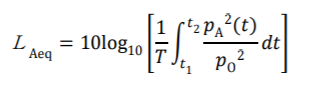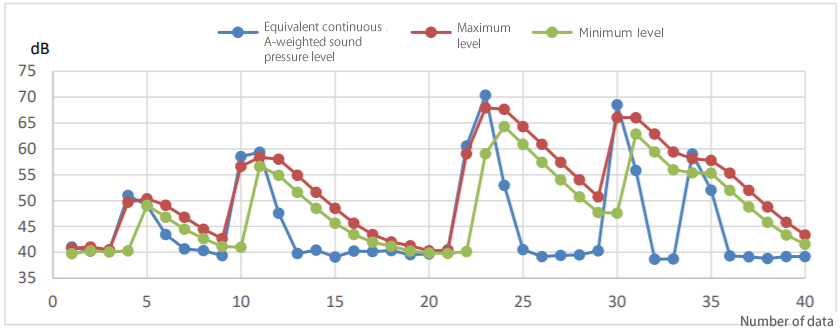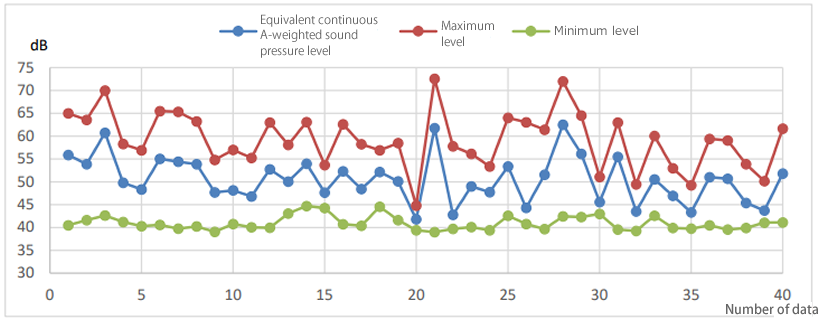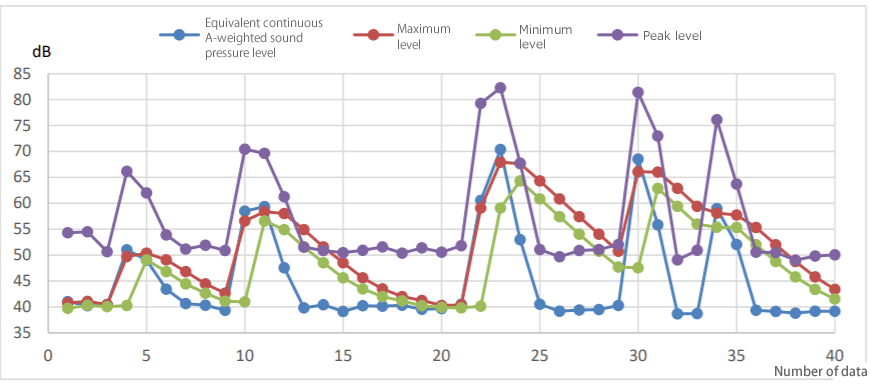Let’s assume to measure repeatedly with the following condition:
Frequency weighting: A, Time-weighted: FAST, Calculation time: 0.1 sec.
The equivalent continuous A-weighted sound pressure level is obtained from the following equation.
(Electroacoustic standard JIS C 1509 Sound level meter)LAeq: Equivalent continuous A-weighted sound pressure level
[The parameter shows the noise level of the steady sound with the energy equivalent to total energy of fluctuating noise for T seconds (from t1 to t2).]

T : Measurement time (t1 to t2)

𝑝A (t) : A-weighted sound pressure

𝑝0 : Reference sound pressure (20 μPa)

Please take note that the above equation is not time-weighted. The LAeq is not affected by the time-weighted setting of the sound level meter.
On the other hand, as the Lmax or the Lmin are time-weighted, the noise level for the short intervals cannot capture the change of the sound pressure level.

Fig.1Fig.2The figure 1 is a plot diagram in which the LAeq with 0.1 sec intervals and the maximum and minimum noise level with the same intervals are measured for 4 seconds. When a sudden sound occurs, the LAeq becomes higher than the maximum, and it becomes lower than the minimum as shown.

The figure 2 is a plot diagram in which the LAeq with 2 sec intervals and the maximum and minimum noise level with the same intervals are measured for 80 seconds. The LAeq is plotted between the maximum and the minimum level, and is averaged as you see.

Since the LAeq is not time-weighted as mentioned above, if it is calculated for a too short period of time, it may deviate from the noise level value with Frequency weighting: A, Time-weighted: FAST (125 ms). It is appropriate to set a few seconds or more interval.

Reference

In some cases, the peak level value 𝐿peak is used as the evaluation value of the sudden sound. The 𝐿peak is the level of the maximum instantaneous sound pressure (AC signals). As the absolute value of the instantaneous sound pressure is taken, it is dependent on frequency weighting, with no relation to time weighting, and is called peak sound level in JIS C1509-1.

The figure 3 shows the 𝐿peak is plotted in the figure 1 in addition. You can see that it has steep rising/falling characteristics compared to the maximum value.

However, since the peak value is not time-weighted, it behaves differently from the noise level.
Please note that the 𝐿peak is effective as an index that indicates whether the measured sound is impulsive or not, however, it is different from the noise level value.

Fig.3(H.O)